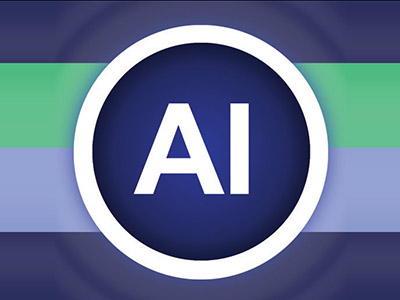ΓραμματείαSecretariat: 2410 684574 | ΦοιτητικάStudents: 2410 684387 g-ds@uth.gr
Select Page

# Artificial Intelligence

Y602

6

#### Hours/Week - ECTS

5 – 5### Learning Outcomes

The course aims at an in-depth understanding of Artificial Intelligence (AI) as it is expressed in its course of development. It covers both Classical (Symbolic) AI, where the problem is described abstractly and standardized with symbols and combined with solving algorithms to solve it, and Computaonal (Computaonal) AI in which complex mathematical models and theories are used, and intelligence is derived through extensive mathematical calculations.

Upon completion of the course, students will be able to:

• understand what “intelligence elements” exist in individual technologies
• describe problems in the state space and solve them with the appropriate search algorithm
• understand the specifics of each search algorithm
• understand the representation of knowledge with rules and the operation of rule-based systems (expert systems, rule systems)
• design genetic algorithms for solving large-scale optimization problems
• understand the concept of vagueness and the mechanisms of fuzzy reasoning and fuzzy control
• understand the basic operation of simple neural networks

### Indicative Module Content

• Classic Artificial Intelligence:
• standardized problem description
• blind search algorithms
• heuristic search algorithms
• algorithms in competitive “games”
• knowledge representation & reasoning
• knowledge systems / expert systems
• Computational Artificial Intelligence:
• Genetic Algorithms
• Fuzzy Logic and Fuzzy Control
• Artificial Neural Networks